# Olympiad Test: Number Ranking - 1

## 10 Questions MCQ Test Mathematical Olympiad Class 8 | Olympiad Test: Number Ranking - 1

Description
Attempt Olympiad Test: Number Ranking - 1 | 10 questions in 20 minutes | Mock test for Class 8 preparation | Free important questions MCQ to study Mathematical Olympiad Class 8 for Class 8 Exam | Download free PDF with solutions
QUESTION: 1

### How many odd numbers are there in the sequence each of which is immediately followed by an odd number? 5 1 4 7 3 9 8 5 7 2 6 3 1 5 8 6 3 8 5 2 2 4 3 4 9 6

Solution: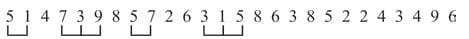QUESTION: 2

### How many odd numbers are there in the sequence which are immediately preceded and also immediately followed by an even number in the sequence? 5 1 4 7 3 2 5 6 8 9 6 7 3 2 1 5 6 4 3 2 7 4

Solution: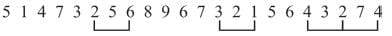QUESTION: 3

### How many even numbers are there which are immediately followed by an odd number and also immediately preceded by an odd number in the sequence? 8 4 7 6 5 3 2 5 1 6 4 3 2 6 7 9 8 5

Solution: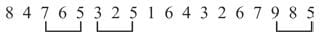QUESTION: 4

In the series
2 5 3 4 8 7 4 2 6 7 1 5 8 3 7 4 5 3
How many pair of successive numbers have a difference of 3?

Solution: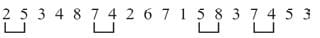QUESTION: 5

How many 5’s are there in the sequence which are immediately preceded by 4 and immediately followed by 7?
2 3 4 5 6 1 4 5 7 1 2 4 5 7 3 8 4 5 9 6 5 3

Solution: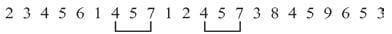QUESTION: 6

How many pair of successive numbers are in the given series whose sum is 12?
4 5 7 3 2 8 4 9 1 5 6 4 8 3 1 9 3 5 7

Solution: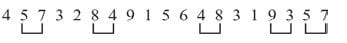QUESTION: 7

How many pair of successive numbers are in the given series whose product is an even number?
6 1 4 6 3 5 1 7 2 6 3 5 7 1 8 3 7 5

Solution: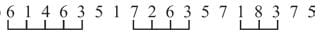QUESTION: 8

How many 8’s are there in the series which are preceded by 5 but not followed by 7?
4 3 8 6 5 8 2 3 5 8 4 7 5 8 7 4

Solution: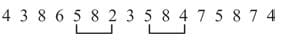QUESTION: 9

How many 9’s are there in the series which are preceded by 7 but not followed by 8?
4 2 9 6 7 9 2 4 7 9 8 4 7 9 3 6 8 9

Solution: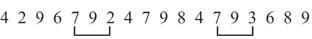QUESTION: 10

What is the product of sum of even digits and sum of odd digits in the number 8 6 7 4 3 5 2?

Solution:

Sum of even digits = 8 + 6 + 4 + 2 = 20
Sum of odd digits = 7 + 3 + 5 = 15
Product = 20 × 15 = 300Use Code STAYHOME200 and get INR 200 additional OFF Use Coupon Code